# RD Sharma Solutions for Class 10 Maths Chapter 9 Arithmetic Progression Exercise 9.5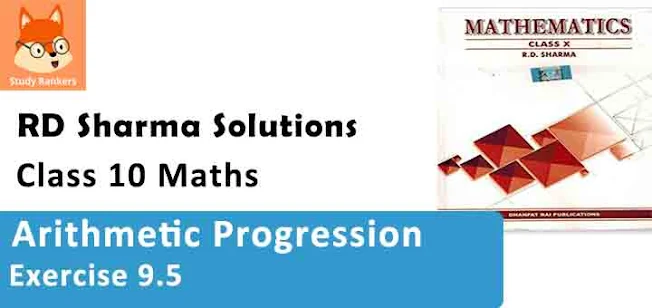1. Find the value of x for which (8x + 4), (6x - 2) and (2x + 7) are in A.P.

Solution

Given,
8x + 4, 6x - 2, 2x + 7 are A.P.
If the given numbers a, b, c are in A.P. then condition is 2b = a + c
Then, 2(6x - 2) = 8x + 4 + 2x + 7
12x - 4 = 10 + 11
⇒ 2x = 15
⇒ x = 15/2

2. If x + 1, 3x and 4x + 2 are in A.P., find the value of x.
Solution
Given numbers,
x + 1, 3x, 4x + 2 are in A.P.
If a, b, c are the given numbers are in AP then, 2b = a + c
Then,
2(3x) = x + 1 + 4x + 2
⇒ 6x = 5x + 3
⇒ x = 3

8. Show that (a – b)2, (a2 + b2) and (a + b)2 are in A.P.
Solution
We have to show, (a – b)2 , (a2 + b2 ) and (a + b)2 are in A.P.
If they are in AP. Then they have to satisfy the condition
2b = a + c
If 2 (a² + b²) = (a – b)² + (a + b)²
If 2 (a² + b²) = a² + b² – 2ab + a² + b² + 2ab
If 2 (a² + b²) = 2a² + 2b² = 2 (a² + b²)
This satisfy the equation which means they are in A.P.

4. The sum of three terms of an A.P. is 21 and the product of the first and the third terms exceeds the second term by 6, find three terms.

Solution

Let the three terms of an A.P. be a – d, a, a + d
Sum of three terms = 21
⇒ a – d + a + a + d = 21
⇒ 3a = 21
⇒ a = 7
and product of the first and 3rd = 2nd term + 6
⇒ (a – d) (a + d) = a + 6
a² – d² = a + 6
⇒ (7)² – d² = 7 + 6
⇒ 49 – d² = 13
⇒ d² = 49 – 13 = 36
⇒ d² = (6)²
⇒ d = 6
Terms are 7-6, 7, 7+6
⇒ 1, 7, 13

5. Three numbers are in A.P. If the sum of these numbers be 27 and the product 648, find the numbers.

Solution

Let the three numbers of an A.P. be a – d, a, a + d
According to the conditions,
Sum of these numbers = 27
a – d + a + a + d = 27
⇒ 3a = 27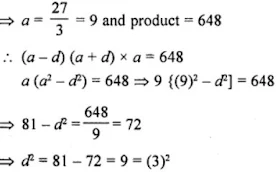⇒ d = 3
Numbers are a-d, a, a+d or, 9-3, 9, 9+3
⇒ 6, 9, 12

6. Find the four numbers in A.P., whose sum is 50 and in which the greatest number is 4 times the least.

Solution

Let the four terms of an A.P. be (a – 3d), (a – d), (a + d) and (a + 3d)
Now according to the condition,
Sum of these terms = 50
⇒ (a – 3d) + (a – d) + (a + d) + (a + 3d) = 50
⇒ a – 3d + a – d + a + d + a – 3d= 50
⇒ 4a = 50
⇒ a = 25/2
and greatest number = 4 x least number
⇒ a + 3d = 4 (a – 3d)
⇒ a + 3d = 4a – 12d
⇒ 4a – a = 3d + 12d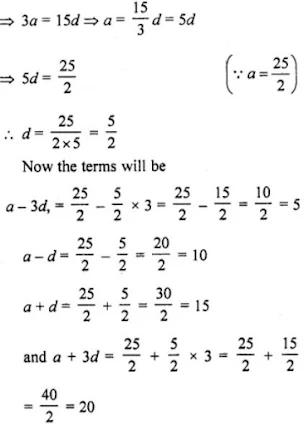The terms are 5, 10, 15, 20.

7. The sum of three numbers in A.P. is 12 and the sum of their cubes is 288. Find the numbers.

Solution

Let the three numbers in A.P. be a-d, a, a+d.
a - d + a + a + d = 12
⇒ 3a = 12
⇒ a = 12/3 = 4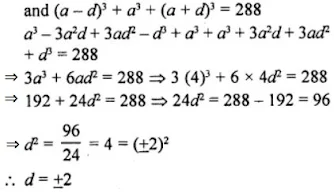Numbers will be 4-2, 4, 4+2 or 2, 4, 6
or, 4+2, 4, 4-2 or 6, 4, 2

8. Divide 56 in four parts in A.P. such that the ratio of the product of their extremes to the product of their means is 5 : 6.

Solution

Let four numbers of A.P. be (a-3d), (A-d), (a+d), (a+3d)
According to question,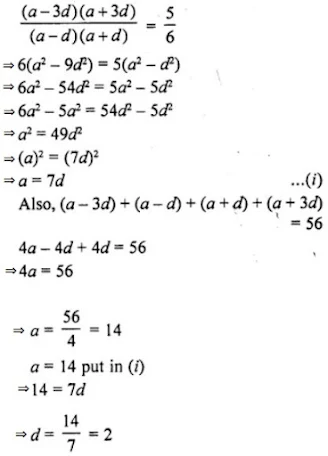Required numbers are:
a - 3d = 14 - 3×2 = 8
a - d = 14 - 2 = 12
a + d = 14 + 2 = 16
a + 3d = 14 + 3×2 = 20
The A.P. is 8, 12, 16, 20.

9. The angles of a quadrilateral are in A.P. whose common difference is 10°. Find the angles.

Solution

Let the four angles of a quadrilateral which are in A.P., be
a – 3d, a – d, a + d, a + 3d
Common difference = 10°
Now sum of angles of a quadrilateral = 360°
a – 3d + a – d + a + d + a + 3d = 360°
⇒ 4a = 360°
⇒ a = 90°
and common difference = (a – d) – (a – 3d) = a – d – a + 3d = 2d
2d = 10°
⇒ d = 5°
Angles will be
a – 3d = 90° – 3×5° = 90° – 15° = 75°
a – d= 90° – 5° = 85°
a + d = 90° + 5° = 95°
and a + 3d = 90° + 3×5° = 90° + 15°= 105°
Hence, the angles of the quadrilateral will ben75°, 85°, 95° and 105°.

10. Split 207 into three parts such that these are in A.P. and the product of the two smaller parts is 4623.

Solution

Let the three parts of the number 207 are (a – d), a and (a + d), which are in A.P.
Now, by given condition,
⇒ Sum of these parts = 207
⇒ a – d + a + a + d = 207
⇒ 3a = 207
a = 69
Given that, product of the two smaller parts = 4623
⇒ a (a – d) = 4623
⇒ 69 (69 – d) = 4623
⇒ 69 – d = 67
⇒ d = 69 – 67 = 2
So, first part = a – d = 69 – 2 = 67,
Second part = a = 69
and third part = a + d = 69 + 2 = 71
Hence, required three parts are 67, 69, 71.

11. The angles of a triangle are in A.P. The greatest angle is twice the least. Find all the angles.

Solution

Given that, the angles of a triangle are in A.P.
Let A, B and C are the angles of a ∆ABC.
2B = A+C ...(i)
We know that, sum of all interior angles of a ∆ABC is 180°
A + B + C = 180°
⇒ 2B + B = 180° [from Eq. (i)]
⇒  3B = 180°
⇒  B = 60°
Let the greatest and least angles are A and C respectively.
A = 2C ...(ii) [by condition]
Now, put the values of B and A in Eq. (i), we get
2×60° = 2C + C
⇒  120° = 3C
⇒  C = 40°
Put the value of C in Eq. (ii), we get
A = 2×40°
⇒ A = 80°
Hence, the required angles of triangle are 80°, 60° and 40°.

12. The sum of four consecutive numbers in A.P. is 32 and the ratio of the product of the first and last terms to the product of two middle terms is 7 : 15. Find the number.

Solution

Let the four consecutive numbers in A.P. be
a - 3d, a - d, a + d, a + 3d
So, a-3d + a-d + a+d + a+3d = 32
⇒ 4a = 32
⇒ a = 8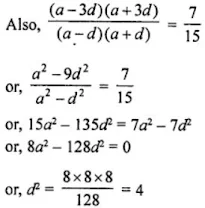or, d = ± 2
So, when a = 8, d = 2, the numbers are 2, 6, 10, 14.